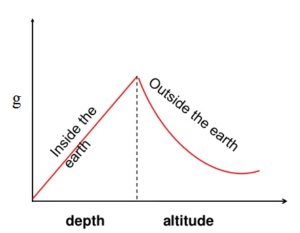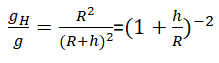# Variation of Acceleration due to Gravity(a)Variation of g due to the shape of the earth

-Since earth is approximately an ellipsoid ( flattened at the poles and bulged at the equator), the equatorial  radius is greater than polar radius by 21 km.
Mathematically,Fig. variation of g due to the shape of the earth

Value of g on the surface of the earth is given byTherefore , value of g is greater in pole than in the equator.

(b)Variation of g due to Height(Altitude)

-The value of acceleration due to gravity decreases with height.[Variation of force of gravity with the distance from the earth]

-Let g  andbe the acceleration due to gravity on the surface of the earth and at height ‘h’ above the surface of the earth respectively .Fig. Acceleration due to gravity at a height 'h'

Acceleration due to gravity on the surface of the earth is given by ,where M is the mass of the earth and R is the radius of the earth,
Similarly, acceleration due to gravity at the height ‘h’ from the surface of the earth is given by,Dividing equation (ii) by (i),Thus value of acceleration due to gravity decreases  with increase in the height above the surface of the earth.Variation of g due to the Depth
-The value of acceleration due to gravity decreases with depth.[When an object is at distance r from the center, only the mass inside a sphere of radius r exerts a net gravitational force on it]

-If a body is taken to a  depth ‘d’ below the earth’s surface , the body will be attracted only by the mass (M’) of the earth which is enclosed by a sphere of radius r=R-d .Fig. Acceleration due to gravity at a depth d

Acceleration due  to gravity at depth ‘d’ is given by the expression,Thus ,the value of acceleration due to gravity decreases with increment in depth below the surface of the earth .

-When d=R we get  g’=0..i.e acceleration due to gravity at the center of the earth is zero.

Comparison of g inside, on and above the earthfig. variation of g inside and outside the earth

-          The value of g linearly increases with the increase in distance from center of earth

-          It attains maximum value at the surface.

-          Above the surface of the earth  , it decreases following  inverse square law.

Variation of g due to Rotation of Earth
-The acceleration due to gravity decreases due to the rotation of the earth.-When the earth is not rotating the weight(W) of an object with mass ‘m’ at a point 'p' on the surface of the earth at latitude of 'φ' is ,

W=mgFig. Variation of g due to the rotation of the earth
The resultant of the force of gravity and  centrifugal  force is given by ,This is the required expression for the variation of acceleration due to gravity due to the rotation of the earth .This equation shows that due to rotation of the earth , the acceleration due to gravity decreases.